# Polynomials-MCQ

MULTIPLE CHOICE QUESTIONS
POLYNOMIALS
1.  The quadratic polynomial whose sum of zeroes is 3 and product of zeroes is –2 is :
(a)   x2 + 3x – 2
(b)   x2 – 2x + 3
(c)   x2 – 3x + 2
(d)   x2 – 3x – 2
2.  If (x + 1) is a factor of 2x3 + ax2 + 2bx + 1, then find the values of a and b given that 2a – 3b = 4
(a)   a = –1, b = –2
(b)   a = 2, b = 5
(c)   a = 5, b = 2
(d)   a = 2, b = 0
3.  The number of zeroes that polynomial f(x) = (x – 2)2 + 4 can have is:
(a)   1
(b)   2
(c)   0
(d)   3
4.  The zeroes of the polynomial f(x) = 4x2 – 12x + 9 are:
(a)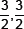(b)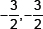(c)   3, 4
(d)   –3, –4
5.  If p(x) is a polynomial of at least degree one and p(k) = 0, then k is known as
(a)   value of p(x)
(b)   zero of p(x)
(c)   constant term of p(x)
(c)   none of these
6.  If p(x) = ax + b, then zero of p(x)
(a)   a
(b)   b
(c)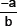(d)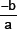7.  Graph of a quadratic polynomial is a
(a)   straight line
(b)   circle
(c)   parabola
(d)   ellipse
8.  Zeroes of a polynomial can be determined graphically. No. of zeroes of a polynomial is equal to no. of points where the graph of polynomial
(a)   intersects y-axis
(b)   intersects x-axis
(c)   intersects y-axis or intersects x-axis
(d)   none of these
9.  If graph of a polynomial does not intersects the x-axis but intersects y-axis in one point, then no, of zeroes of the polynomial is equal to
(a)   0
(b)   1
(c)   0 or 1
(d)   none of these
10.  A polynomial of degree n has
(a)   only 1 zero
(b)   exactly n zeroes
(c)   atmost n zeroes
(d)   more than n zeroes
11.  If p(x) = ax2 + bx + c, then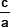is equal to
(a)   0
(b)   1
(c)   sum of zeroes
(d)   product of zeroes
12.  If p(x) = ax2 + bx + c, then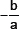is equal to
(a)   0
(b)   1
(c)   product of zeroes
(d)   sum of zeroes
13.  If p(x) = ax2 + bx + c, and a + b + c = 0, then one zero
(a)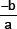(b)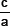(c)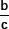(d)   none of these
14.  If p(x) = ax2 + bx + c and a + c = b, then one of the zeroes is
(a)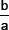(b)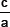(c)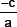(d)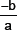15.  A quadratic polynomial whose one zero is 6 and sum of the zeroes is 0, is
(a)   x2 – 6x + 2
(b)   x2 – 36
(c)   x2 – 6
(d)   x2 – 3